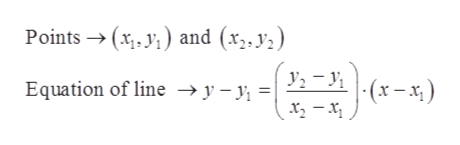# Write an equation of the line through the points (1, 8) and (2, −4). Write the answer in slope-intercept form.

Question
1 views

Write an equation of the line through the points (1, 8) and (2, −4). Write the answer in slope-intercept form.

check_circle

Step 1

Given, points are

Step 2

The equation of line thro...help_outlineImage TranscriptionclosePoints → (x;, y;) and (x,, y2) Equation of line → y – y, = | -(x - x; ) (*-x)- fullscreen

### Want to see the full answer?

See Solution

#### Want to see this answer and more?

Solutions are written by subject experts who are available 24/7. Questions are typically answered within 1 hour.*

See Solution
*Response times may vary by subject and question.
Tagged in

### Equations and In-equations